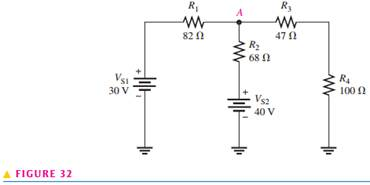### Create an Account

Already have account?

### Forgot Your Password ?

Home / Questions / Write the node voltage equations for Figure 29 Use your calculator to find the node voltages

# Write the node voltage equations for Figure 29 Use your calculator to find the node voltages

Write the node voltage equations for Figure 29. Use your calculator to find the node voltages.Aug 06 2020 View more View Less

#### Answer (Solved)Subscribe To Get Solution